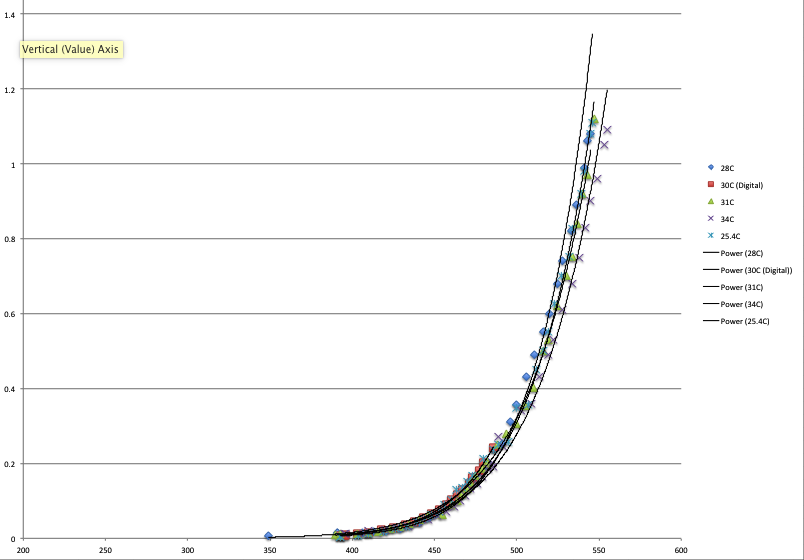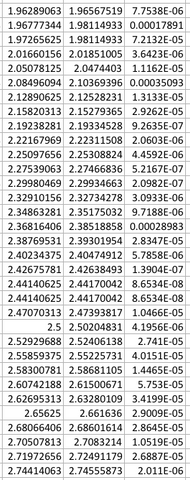# Calibrating The Rev. P Wind Sensor From A New Regression

We reprocessed some old data to add some software temperature compensation for the Rev. P wind sensor. The sensor itself has hardware temperature calibration built in, but the hardware compensation isn’t perfect.You can see by these trend lines in this ADCunit vs static pressure graph that the curves diverge slightly at the upper end of the graph. I used the static pressure data from a pitot tube along with humidity and temp data, to convert the pitot tube data to wind velocities. I then set up a regression and derived an equation that matched the curve of the sensor.

I did the regression, solving for the output voltage instead of the wind speed, as I probably should have done. When the regression was done I had to factor the final equation, solving for the wind speed (in MPH) instead of for the volts, which is what the sensor outputs. This resulted in slightly less clear math, than it might have been, had I done the regression the other way around. I’m far from an expert Excel jockey, but knowing how to use the “Solver” in Excel makes me feel like at least I could play one on TV, after maybe a clean up and a shave.

The Solver!Just so that I can show you what a work of inspiration Excel’s Solver is, take a look at the data on the left. The two left hand columns represent the actual measured voltage values in one column and the calculated values in the other – from the generated equation. The rightmost third column is the difference between the two columns, squared, to get rid of any minus signs. As you can see, the two columns match each other very closely, confirming that the equation generates curves that are very close to the curves actually generated by the sensor. Wind speeds in the data ranged from zero to about 50 MPH.

By the way, these errors are how the Solver actually works. The solver manipulates the constants in the equation below to try to minimize the summed errors across all of the temperature series (there were 4 temperature data points spanning 7.7C).

Of course it’s always possible, that with a wider range of data, the form of my equation would break down, and the curves would start diverging from the actual measured data. In that case I would have to introduce an equation with more terms for a more sophisticated ability to capture the actual shape of all the data.

Here’s the equation derived from the regression for the Rev P wind sensor.

WS_MPH = (((Volts – ZeroWind_V) / (3.038517 * (Temp_C ^ 0.115157 ))) / 0.087288 ) ^ 3.009364

The terms are:
WS_MPH: wind speed in miles per hour
Volts: the output of the Wind Sensor Rev. P at the “Out” pin in volts
Temp_C: temperature in degrees C
ZeroWind_V: zero wind voltage – measured with the sensor angled at the edge of a table with a glass over the tip (loop etc.) of the sensor. Let the sensor stabilize for 40 seconds or so, when the voltage stops dropping and stabilizes, record the voltage.

You may just substitute 1.3692 for the zero wind term, which is the figure that I used for the regression, or else measure your own sensor’s zero wind voltage and use that voltage. 1.3692 volts is the data I had for zero wind from the original measurements. I measured 8 of our current wind sensor’s zero wind voltage and ended up with the following chart.

1.336 volts
1.332
1.341
1.337
1.327
1.356
1.380
1.361

which average to 1.346. You should see a similar voltage when measuring with the technique above or your sensor is malfunctioning. This is also at room temperature of around 25 degrees C. It seems that our current production has a slightly lower value than this old data. We did make some small changes in production with the Rev. P wind sensor, to move to .1% resistors for selected parts. 0.1% resistors are not stocked in small quantities in every value, so we had to adjust some values, keeping the ratios as close as we could.

You’ll need to code this up in C. Most people will be using microcontrollers with ADC’s so you’ll need to input the reference voltage of your microcontroller and the bit depth of the ADC. For example with an Arduino UNO or Mega running at 5.0 volts, which have a 10-bit ADC, you would substitute the following expression for the term “Volts”  “(float)analogRead(A0) * 5.0 / 1023.0”

The temp sensor on the board is a Microchip MCP9701AT. The datasheeet describes the transfer equation as

VOUT = TC x TA + V0°C.

Where:
TA = Ambient Temperature
VOUT = Sensor Output Voltage
V0°C = Sensor Output Voltage at 0°C
TC = Temperature Coefficient

We will need to rearrange this a tad as the Vout is known and Tambient is the term for which we wish to solve.

So rearranging we have

Tambient = ( Vout – V0°C ) / TC

Checking the datasheet,
TC, temperature coefficient for our part is 19.5 mV/°C
V0°C, the voltage at zero degrees C is 400 mV

So our equation becomes:

Tambient = ( Vout – 0.400 ) / 0.0195

Just for a sanity check I  checked a couple of sensors and ran the values through the equation, resulting in 25.0 C and 25.7 C – in a comfortable air conditioned office on a sunny day in August. Close enough to be relatively accurate. You can just substitute this expression for the TempC value in the regression above. You will need to read your ADC and convert it to volts however, similar to the expression above for the “Volts” output.

Caveats:
What is life without some “ifs, ands and buts”? The temp data that I did the regression from only included a temp range of 7.7 degrees C. and 34 was as hot as it got. So the regression is bound to be somewhat inaccurate at lower temperatures. Luckily temperature doesn’t affect the output that much so it probably will not affect the usefulness of the sensor that much. However as I said above, the sensor may have had a slight revision since the original data was captured, resulting in other possible inaccuracies. Ideally one would wish for a much wider temperature range and a much wider range of wind speeds. The data in the regression goes up to about 50 MPH. There is probably enough information in the data for the curves to be able to extend the measurements somewhat, but the farther you get from 25C in temperature (and especially cold) and above 50 MPH, the more error there is likely to be in the equation above.